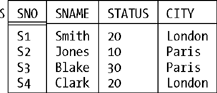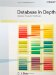# Relations Versus Relvars

 Now, it's entirely possible that you already knew everything I've discussed in this chapter so far; in fact, I rather hope you did (though I also hope that doesn't mean you found the discussions boring). Anyway, now I come to something you might not know already. The fact is, historically there's been a lot of confusion between relations I mean relations as such on the one hand, and relation variables on the other. Forget about databases and relations for a moment; instead, consider the following simple programming language example. Suppose I say in some arbitrary programming language: ` DECLARE N INTEGER ... ;` Then N here is not an integer. Rather, it's a variable whose values are integers as such (different integers at different times). Right? I'm sure we can agree on that. Well, in exactly the same way, if we say in SQL: ` CREATE TABLE T ... ;` then T is not a table; it's a table variable or (as I would prefer to say, ignoring various SQL quirks such as nulls and duplicate rows) a relation variable, whose values are relations as such (different relations at different times). Consider Figure 1-3 once again. That figure shows three relation values: namely, those that happen to appear in the database at some particular time. But if we were to look at the database at some different time, we would probably see three different relation values appearing in their place. In other words, S, P, and SP in that database are really variables: relation variables, to be precise. For example, suppose the relation variable S currently has the value the relation value shown in Figure 1-3, and suppose we delete the tuples (actually there's only one) for suppliers in Athens: ` DELETE S WHERE CITY = 'Athens' ;` Here's the result:Conceptually, what's happened is that the old value of S has been replaced in its entirety by a new value. Of course, the old value (with five tuples) and the new one (with four) are very similar, but they certainly are different values. In fact, the DELETE just shown is logically equivalent to, and indeed shorthand for, the following relational assignment:[*][*] I can't show this in SQL because SQL doesn't directly support relational assignment. Throughout this book, I'll show examples in SQL wherever possible but when it's not possible for some reason, as here, I'll use a more or less self-explanatory (and truly relational) language called Tutorial D instead. Tutorial D is the language Hugh Darwen and I use to illustrate relational ideas in our book Databases, Types, and the Relational Model: The Third Manifesto, Third Edition (Addison-Wesley, 2006); you can regard it as a realization in concrete syntax of the abstract constructs of the relational model (which SQL, regrettably, is not). ` S := S WHERE NOT ( CITY = 'Athens' ) ;` As with all assignments, the effect here is that (a) the source expression on the right side is evaluated, and (b) the result of that evaluation is then assigned to the target variable on the left side, with the overall result already explained. In like fashion, of course, the familiar INSERT and UPDATE statements are also really shorthand for certain relational assignments. Thus, as I mentioned in the section "A Review of the Original Model," relational assignment is the fundamental update operator in the relational model, and indeed it's the only update operator that's really needed, logically speaking. So we have these two different concepts, relation value and relation variable. The trouble is that the literature has historically used the same term, relation, to stand for both, and that practice has certainly led to confusion. In this book, therefore, I'll distinguish very carefully between the two from this point forward I'll talk in terms of relation values when I mean relation values and relation variables when I mean relation variables. However, I'll also abbreviate relation value most of the time to just relation (exactly as we abbreviate integer value most of the time to just integer). And I'll abbreviate relation variable most of the time to just relvar; for example, I'll say the suppliers-and-parts database contains three relvars. As an exercise, you might like to go back over the text of this chapter so far and see exactly where I used the term relation when I really ought to have used the term relvar instead (or as well).Database in Depth: Relational Theory for Practitioners
ISBN: 0596100124
EAN: 2147483647
Year: 2006
Pages: 127
Authors: C.J. Date

Similar book on Amazon